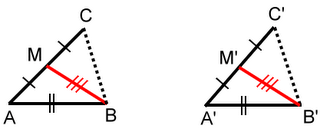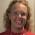### Prove two triangles are congruent

Here's an example geometry problem from Kiseleve's geometry book that I blogged about earlier. This problem is not difficult and is a good example of problem where you need to prove triangles congruent.

The reason I'm solving it here is simply to help those of you who will be homeschooling your child thru high school geometry. It helps enormously to be familiar with proofs and the type of reasoning before you actually need to write proofs yourself.

Problem:

80. Prove that if two sides and the median drawn to the ﬁrst of them in one triangle are respectively congruent to two sides and the median drawn to the ﬁrst of them in another triangle, then such triangles are congruent.

First draw a picture. We have two triangles with certain parts congruent, and we are supposed to prove that the triangles are congruent.We know that AB = A'B', AC = A'C', and BM = B'M' (givens).

This proof in a nutshell is based on first proving that the two triangles AMB and A'M'B' and congruent (by SSS), from which follows that angle BAC = angle B'A'C'. Then the original triangles are congruent by SAS.

Consider now the two triangles AMB and A'M'B'. We already know two of their corresponding sides are congruent - AB = A'B', and BM = B'M'. But even their third sides are congruent, because by the fact M is a midpoint of AC, AM = 1/2AC and similarly A'M' = 1/2A'C'. Since AC and A'C' are congruent, it follows that half of those line segments, or AM and A'M' are congruent also.

By the SSS congruence theorem (or postulate; if your book calls it that) for triangles, the triangles AMB and A'M'B' are congruent.

Therefore their corresponding angles are congruent also. In particular, the angle BAC = angle B'A'C'.

So we have side AB, angle BAC, and side AC congruent to the side A'B', angle B'A'C', and side A'C', respectively, in the other triangle.

Now it follows by the SAS congruence theorem that the two triangles are congruent.

Tags: , , ,

### CommentsHi
I am a student from I.R.IRAN.
I enjoed from your posts.
see my weblog:
http://engmmajidee.blogfa.comAnonymous said…
I can't believe nobody commented on the error in this proof. M is the midpoint of AC not AB. So you have to replace "B" with "C" in the corresponding statements in the proof... Wow, this was posted more than 3 years ago and NOBODY caught it???Maria Miller said…
Anonymous -- it's been fixed, thanks.